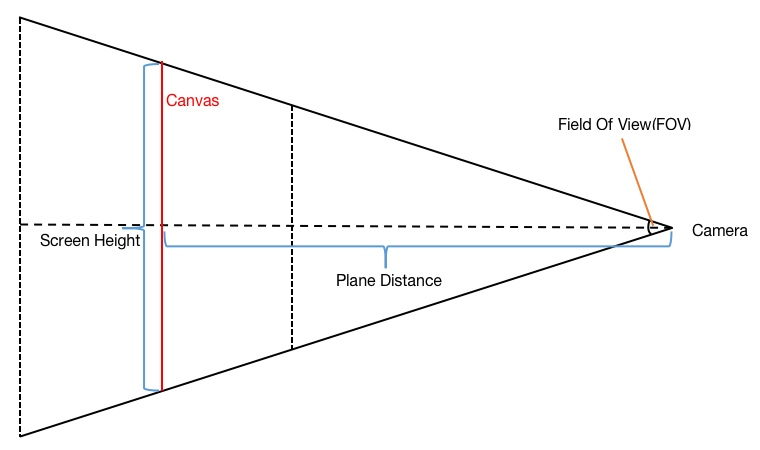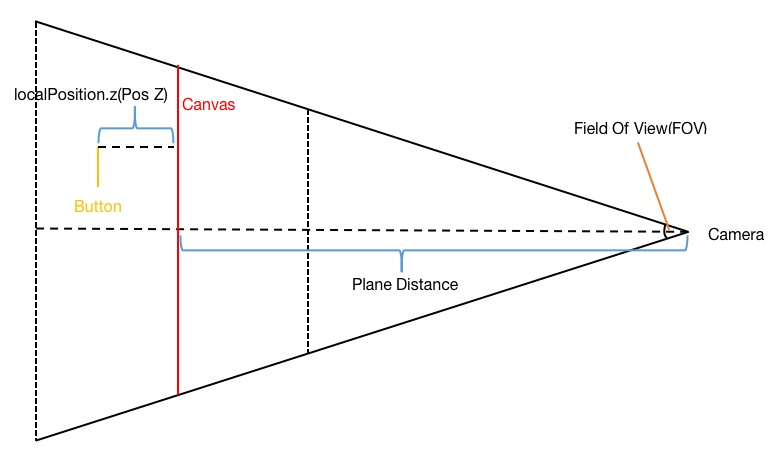# 简单实现这个需求

3D 模型渲染到已有的 Unity UI 上面，使用 Camera、Render Texture 和 Raw Image 来实现；脚本控制模型运动效果，Camera 来拍摄这些效果并渲染到 Render Texture 上，再利用 Raw Image 将 Render Texture 在 Unity UI 层展示出来。

# Screen Space (屏幕空间)到 World Space (世界空间)

1. 当没有相机渲染 Canvas (Canvas 的 Render Mode 设置为 Screen Space - OverlayScreen Space - Camera 且不设置相机)时:

• Canvas 左下角位于世界空间下的原点处(Canvas 此模式下世界空间下 Z 坐标为 0)；

• 位于 Canvas 中的 RectTransform 世界坐标就是其所在的屏幕坐标(X 和 Y 方向)，Z 方向世界坐标是其相对于父元素世界坐标偏移 localPosition.z (或 Pos Z)的值；

2. Canvas 的 Render Mode 设置为 Screen Space - Camera 并设置相机)时:

• Unity 中一个 UI 单位不再是对应一个 Unity 单位；

• Canvas 的 Pivot 处 X 和 Y 方向世界坐标和渲染 Camera X 和 Y 方向世界坐标相等；

• Canvas 所在平面 Z 方向世界坐标由其设置的 Plane Distance 值和渲染 Camera 的 Z 方向世界坐标共同决定；

3. Canvas 的 Render Mode 设置为 World Space 时:

• 此时会将 Unity UI 当做 3D 物体来渲染，Canvas 可以位于空间中任何位置；

• 位于 Canvas 中的 RectTransform 世界坐标即可根据 Canvas 位置以及其 localPosition 得出；

$PerUIUnitUnityUnit = \sum_{n=1}^N Parent(n).Scale$

## 对于 Canvas 的 Render Mode 为 Screen Space - Overlay 或 Screen Space - Camera 且不设置相机 这种模式，Canvas 下 RectTransform 的世界坐标表达式为:

$\begin{eqnarray*} World.Position.x & = & Screen.Position.x \\ World.Position.y & = & Screen.Position.y \\ World.Position.z & = & \sum_{n=1}^N (Parent(n).LocalPosition.z \times PerUIUnitUnityUnit(n)) \\ && + Self.LocalPosition.z \times PerUIUnitUnityUnit(Self) \end{eqnarray*}$

## 对于 Canvas 的 Render Mode 为 World Space，Canvas 下 RectTransform 的世界坐标表达式为:

$\begin{eqnarray*} World.Position.x & = & \sum_{n=1}^N (Parent(n).LocalPosition.x \times PerUIUnitUnityUnit(n)) \\ && + Self.LocalPosition.x \times PerUIUnitUnityUnit(Self) \\ && + Canvas.Position.x \end{eqnarray*}$

$\begin{eqnarray} World.Position.y & = & \sum_{n=1}^N (Parent(n).LocalPosition.y \times PerUIUnitUnityUnit(n)) \\ && + Self.LocalPosition.y \times PerUIUnitUnityUnit(Self) \\ && + Canvas.Position.y \end{eqnarray}$

$\begin{eqnarray*} World.Position.z & = & \sum_{n=1}^N (Parent(n).LocalPosition.z \times PerUIUnitUnityUnit(n)) \\ && + Self.LocalPosition.z \times PerUIUnitUnityUnit(Self) \\ && + Canvas.Position.z \end{eqnarray*}$

## 对于 Canvas 的 Render Mode 为 Screen Space - Camera 并设置相机)，这种情况相对复杂一点。$tan(\frac{FOV}{2}) = \frac{Screen Height \div 2}{Plane Distance}$

$PerUnitPixels = \frac{Screen Height \div 2}{Plane Distance \times tan(\frac{FOV}{2})}$$CanvasPivot.Position.x = Camera.Position.x$

$CanvasPivot.Position.y = Camera.Position.y$

$CanvasPivot.Position.z = Camera.Position.z + Plane Distance$

Canvas 的 Pivot 世界坐标最终表达式:

$\begin{eqnarray*} CanvasPivot.Position & = & (Camera.Position.x, Camera.Position.y, \\ && Camera.Position.z + Plane Distance) \end{eqnarray*}$

$\begin{eqnarray*} RectTransform.Position.z & = & \frac{\sum_{n=1}^N Parent(n).LocalPosition.z + RectTransform.LocalPosition.z}{PerUnitPixels} \\ && + CanvasPivot.Position.z \end{eqnarray*}$

$\begin{eqnarray*} RectTransform.Position.z & = & (\sum_{n=1}^N (Parent(n).LocalPosition.z \times PerUIUnitUnityUnit(n)) \\ && + RectTransform.LocalPosition.z \times PerUIUnitUnityUnit(RectTransform)) \\ && \div PerUnitPixels \\ && + CanvasPivot.Position.z \end{eqnarray*}$

RectTransform 世界坐标最终表达式如下:

$\begin{eqnarray*} RectTransform.Position & = & (\sum_{n=1}^N (Parent(n).LocalPosition \times PerUIUnitUnityUnit(n)) \\ && + RectTransform.LocalPosition \times PerUIUnitUnityUnit(RectTransform)) \\ && \div PerUnitPixels \\ && + CanvasPivot.Position \end{eqnarray*}$